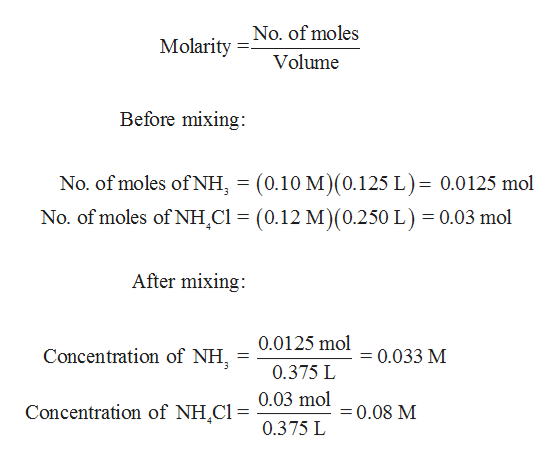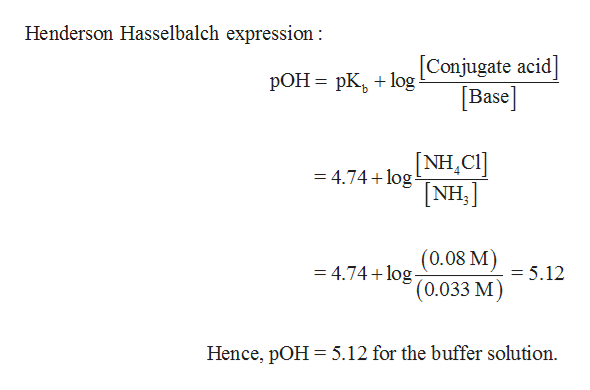# Calculate the pH of a buffer obtained by mixing 125 mL of 0.10 M NH3 with 250 mL of 0.12 M NH4Cl. Kb of NH3=1.8 x 10-5.

Question
14 views

Calculate the pH of a buffer obtained by mixing 125 mL of 0.10 M NH3 with 250 mL of 0.12 M NH4Cl. Kb of NH3=1.8 x 10-5.

check_circle

Step 1

pKb of the buffer solution has to be determined for calculating the pOH of the solution.

Step 2

Concentration of 0.10 M NH3 and 0.12 M NH4Cl in the solution is determined using the formula form molarity. Total volume of the solution after mixing is 125 mL + 250 mL = 375 mL = 0.375 L.

1 mL = 0.001 Lhelp_outlineImage TranscriptioncloseNo. of moles Molarity Volume Before mixing No. of moles of NH, (0.10 M)(0.125 L)= 0.0125 mol No. of moles of NH Cl (0.12 M) (0.250 L) 0.03 mol After mixing: 0.0125 mol Concentration of NH =0.033 M 0.375 L 0.03 mol Concentration of NH,Cl= = 0.08 M 0.375 L fullscreen
Step 3

pOH of the solution can be determined usi...help_outlineImage TranscriptioncloseHenderson Hasselbalch expression Conjugate acid Base pOH pKog [NH,CI] NH, = 4.74 log (0.08 M) (0.033 M) = 4.74 log = 5.12 Hence, pOH 5.12 for the buffer solution fullscreen

### Want to see the full answer?

See Solution

#### Want to see this answer and more?

Solutions are written by subject experts who are available 24/7. Questions are typically answered within 1 hour.*

See Solution
*Response times may vary by subject and question.
Tagged in

### Equilibrium Concepts